# Combine Multiple Worksheets into One

In this article, we are going to learn how to assemble the data from different workbooks through VBA in Microsoft Excel.

Let’s understand with a simple exercise, how to merge spread sheet through VBA in Microsoft Excel.

We have 3 different Excel files. 2 files are to xlsx and one file is to xlsm in a folder. We want to collate both data sets in Excel file to keep few things in mind that in every data, rows and columns can be increase and decrease, and also we don’t know the Excel file names and how many files are there in a folder.

In this sheet, we want to combine the data:-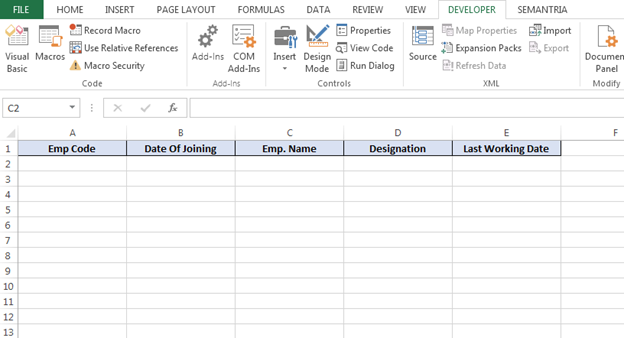1st Data:-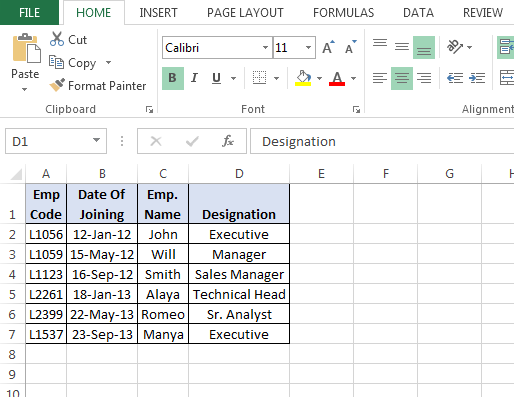2nd Data:-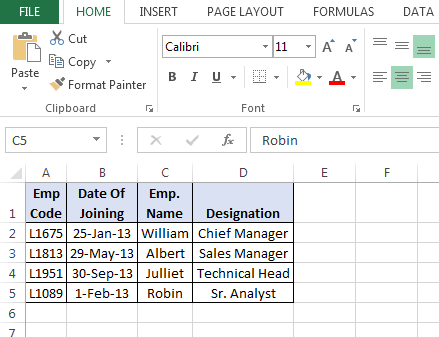3rd Data:-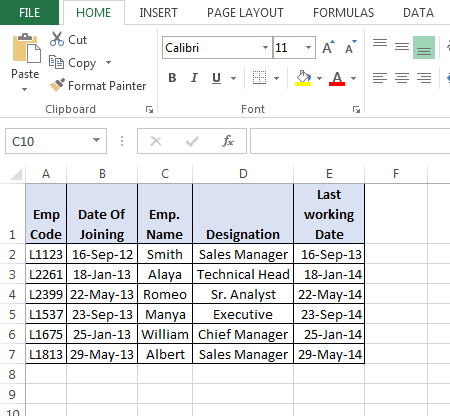Now, we want to collate the data in a sheet. We need to follow below given steps and code:-

• Press the key Alt+F11 to open the VBE page to write the macro.
• Then go to insert tab and insert a module.
• Write below mentioned code in the page.

Sub Collate_Data()

Dim FolderpathAs String, filePath As String, Filename As String

Folderpath = "E:\Excel Tips\New VBA topics\HR Data\"      ‘(mention the file path)

filePath = Folderpath& "*xls*"

Filename = Dir(filePath)

Dim LastrowAs Long, Lastcolumn As Long
Do While Filename <> ""

Workbooks.Open (Folderpath& Filename)

Lastrow = ActiveSheet.Cells(Rows.count, 1).End(xlUp).Row

Lastcolumn = ActiveSheet.Cells(1, Columns.count).End(xlToLeft).Column

Range(Cells(2, 1), Cells(Lastrow, Lastcolumn)).Copy

ActiveWorkbook.Close

erow = Sheet1.Cells(Rows.count, 1).End(xlUp).Offset(1, 0).Row

ActiveSheet.Paste Destination:=Worksheets("Sheet1").Range(Cells(erow, 1), Cells(erow, 5))

Filename = Dir

Loop

End Sub

Code explanation: - Firstly, we will define the all variable which we need to use while describing the code. Then we will use Do While loop in code to run the program for all Excel files. Then we will define the path of files, and after that we will define the last row and column in Excel sheet and then we will define the destination to paste the data.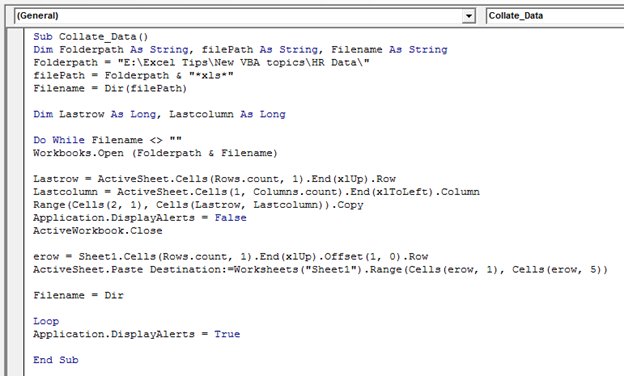• To run the macro, press the key F5.
• Data of both sheets will be copied and pasted in an Excel sheet.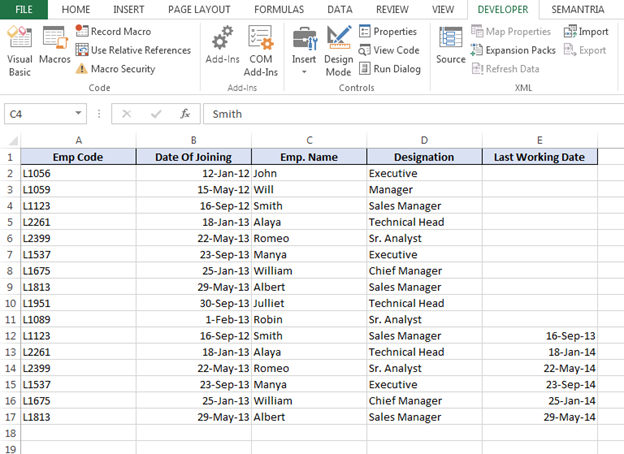This is the way we can collate the data in a sheet from different files of Excel through VBA in Microsoft Excel.

1.I want to combine Multipal workbook sheets in one master sheet

•I'll be back with the code for you.

2.Doesn't work. I had 3 files and wanted to combine them in one sheet. This code takes only one file name (doesn't rotate through all the files). So, the output is - no changes done.

3.the above code doesn't work exactly as you mention
syntax error

Sub Collate_Data()
Dim FolderpathAs String, filePath As String, Filename As String
filePath = Folderpath& "*xls*"
Dim LastrowAs Long, Lastcolumn As Long
Workbooks.Open (Folderpath& Filename)

4.the above code doesn't work exactly as you mention. code copy data from all workbooks but put second sheet's data over the first sheet's data. so you lose rows. can you help me with this?

5.Hi ,

Thanks for the Macro its works fine but when i am copying data it tend to change date format for example( from 05/09/2018 it changes to 09/05/2018) wielder it is doing on some rows only.

Any Idea ??